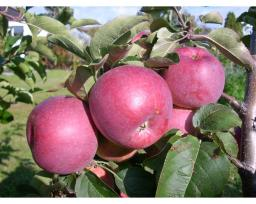# Individual 5028

Mrs. Bartova is buying apples. The Bohemia variety is CZK 4 more expensive per kilogram than the Golden variety. Mrs. Bartova bought 10 kg of Golden and 15 kg of Bohemia. She paid a total of 360 kc. How many crowns did the individual types of apples cost?

b =  16 Kc/kg
g =  12 Kc/kg

### Step-by-step explanation:

b=4+g
10g + 15b = 360

b=4+g
10·g + 15·b = 360

b-g = 4
15b+10g = 360

Pivot: Row 1 ↔ Row 2
15b+10g = 360
b-g = 4

Row 2 - 1/15 · Row 1 → Row 2
15b+10g = 360
-1.67g = -20

g = -20/-1.66666667 = 12
b = 360-10g/15 = 360-10 · 12/15 = 16

b = 16
g = 12

Our linear equations calculator calculates it.Did you find an error or inaccuracy? Feel free to write us. Thank you!

Tips for related online calculators
Do you have a linear equation or system of equations and looking for its solution? Or do you have a quadratic equation?